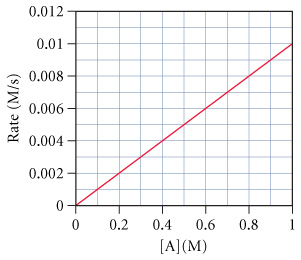# Problem: The graph below shows a plot of the rate of a reaction versus the concentration of the reactant A for the reaction:A → products .Estimate the value of k.

###### FREE Expert Solution

We’re being asked to calculate the rate constant with the correct units for the given reaction. We can calculate the rate constant of the reaction from its rate law.

Recall that the rate law only focuses on the reactant concentrations and has a general form of:

k = rate constant
A & B = reactants
x & y = reactant orders

83% (131 ratings)###### Problem Details

The graph below shows a plot of the rate of a reaction versus the concentration of the reactant A for the reaction:

A → products.

Estimate the value of k.